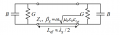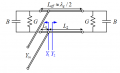# apparture theory conflicts with antenna gain theory in MSA

#### Tesla23

Joined May 10, 2009
484
You need to unpack this - there are several things going on:

The gain (ignoring losses) is set purely from the antenna pattern. The H-plane pattern gets narrower as the patch width increases by simple fourier transform theory applied to the length (W) of the slot radiator. The E-plane pattern is dominated by the array pattern of the two effective slot antennas (one at each end of the patch). Note that for the er=1 case that the patch is about half a free-space wavelength long (as we would expect), so the two slot elements are about half a wavelength spaced. This gives more gain than the higher er cases where the patch is shorter (the er=9.8 case has a spacing of about lambda/6). Just look at the directivity of a two element array with a spacing of lambda/2 compared to lambda/6.

The bandwidth is determined by the loading of the radiation resistance on the microstrip resonator. The microstrip transmission line for all these patches has a characteristic impedance around 8.5 ohms (presumably the width of the patches was chosen, as er changed, to keep this about constant). So for the same voltage at the ends of the patches, there will be roughly equivalent stored reactive energy in the microstrip tx line, so as the radiation conductance increases as W increases, you would expect the Q to decrease as W increases. Q has no direct influence on the gain.

#### yef smith

Joined Aug 2, 2020
293
Hello Tesla, the last sentense is the most problematic for me.

"so as the radiation conductance increases as W increases"
bandwidth meens that our input impedance in the frequency range is the closest to 50 ohm on a smth chart.
so given characteristic impedance around 8.5 ohms .
How exactly radiation resitance will make us closer to 50 ohms in a wider frequency range?
Thanks.

#### Tesla23

Joined May 10, 2009
484
This comes from the simple transmission line model of the patch:All these patches have approximately the same line impedance, all that is changing is the increasing G as W increases. (B also increases with W, but this simply alters the length of the resonator slightly for resonance.) You should be able to work out the Q of the transmission line resonator above and show that as G increases, Q decreases (to simplify matters, do the analysis with B=0 and L=λg/2). The radiation conductances G are typically smaller than 1/50Ω, hence at resonance you can match to 50Ω by simply moving the feedpoint in from the edge:This is all basic transmission line theory, much simpler than the problem of working out the size of G for example.

This seems to be explained fairly well here: https://www.ece.mcmaster.ca/faculty/nikolova/antenna_dload/current_lectures/L21_Mstrip2.pdf, which is where I pasted the diagrams from.